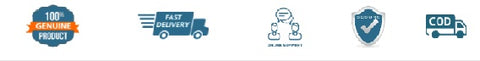# Mathematics for Class 8 (R.D. Sharma) - 2023-24

SKU:3M_Dhanpat_28049
Price : Rs. 582.00
Sale price Rs. 582.00 Price : Rs. 645.00
Sold out
Unit price
per
Shipping calculated at checkout.

The sincere effort and constant struggle of the author (S) has made it possible for him to introduce new concepts in a simple language so that children are able to grasp them easily. Using attractive illustrations, basic concepts have been explained in a concise manner so that students find them interesting and easy to understand. The revised edition is based on the latest syllabus outlined by National Council of education research and Training (NCERT) for the students of Class 8. The book has been categorised under 27 chapters, where the content is written in a lucid language. Both easy and tough problems are given to encourage the students to practice more and more so that they become confident in solving the exercises. Chapters included in the textbook rational numbers powers squares and Square Roots Cubes and Cube Roots playing with Numbers algebraic Expressions and Identities factorization division of algebraic Expressions linear equation in one variable direct and Inverse variations time and Work Percentage Profit, Loss, discount and Value added tax (VAT) Compound Interest understanding shapes-i (Polygons) understanding shapes-ii (Quadrilaterals) understanding shapes-iii (special types of Quadrilaterals) practical Geometry (construction) Visualising shapes Mensuration-I (area of a trapezium and a Polygon) Mensuration-II (volumes and Surface areas of a cuboid and a Cube) mensuration-iii (surface Area and Volume of a right circular cylinder) data handling-i (classification and tabulation of data) data handling-ii (graphical representation of data as histograms) data handling-iii (pictorial representation of data as pie charts or circle graphs) data handling-iv (probability) introduction to graphs salient features of the textbook mathematical concepts have been explained in the easiest way. Each br>Chapter has a good number of illustrative Examples to comprehend the applications of basic concepts. In all the chapters, illustrative examples, followed by an exercise containing numerous unsolved problems, have been provided.## Customer Reviews

Based on 1 review
100%
(1)
0%
(0)
0%
(0)
0%
(0)
0%
(0)
R
R.D. GHRITLAHRE